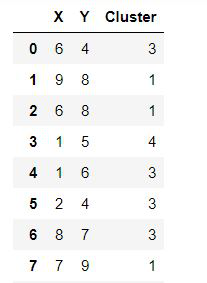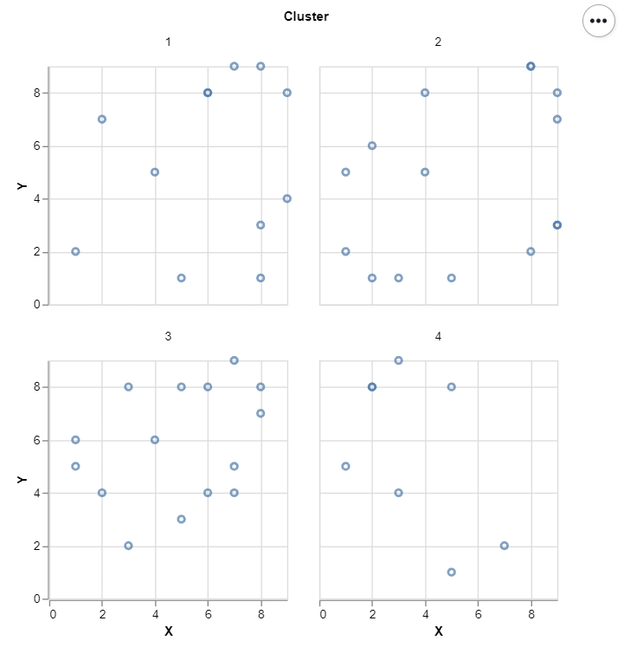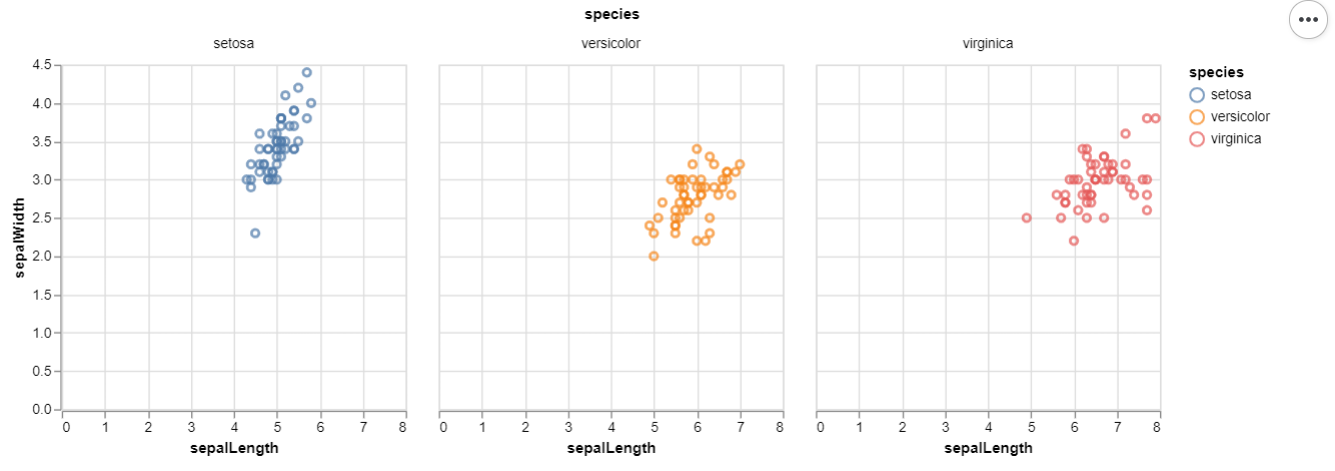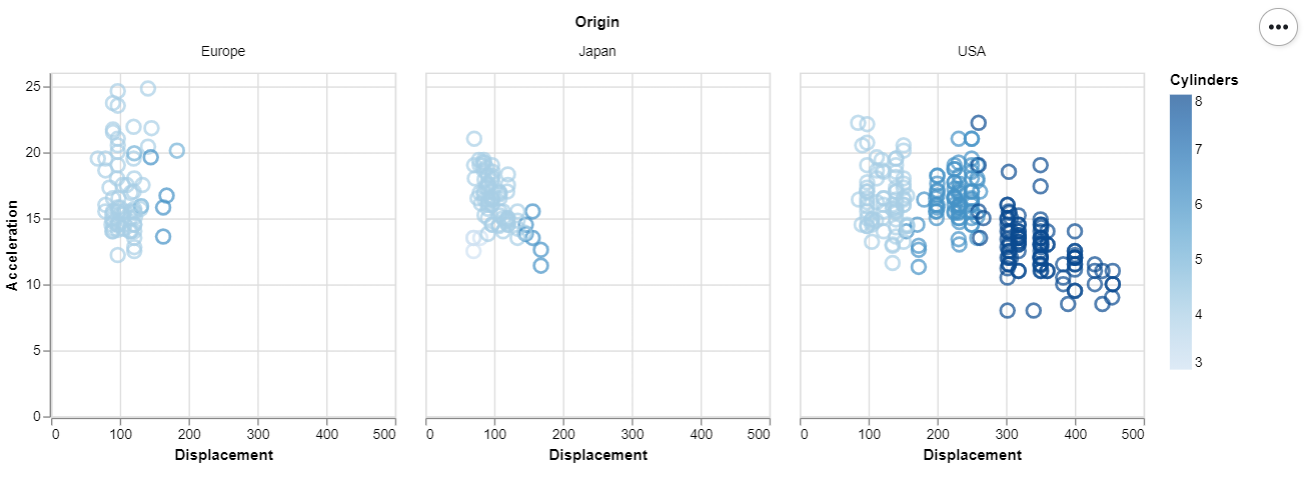Related Articles

# How To Facet a Scatter Plot with Altair?

• Last Updated : 03 Jan, 2021

In this article, we will learn how to Facet a Scatter Plot with Altair. Let’s recall some concepts :

• Altair is a statistical visualization library in Python. It is declarative in nature and is based on Vega and Vega-Lite visualization grammars. It is fast becoming the first choice of people looking for a quick and efficient way to visualize datasets. If you have used imperative visualization libraries like matplotlib, you will be able to rightly appreciate the capabilities of Altair.
• A scatter plot (also called a scatterplot, scatter graph, scatter chart, scattergram, or scatter diagram) is a type of plot or mathematical diagram using Cartesian coordinates to display values for typically two variables for a set of data.

Here, we are making the scatter plot using Altair library. For this, we use Chart() function in Altair to load the data and then use the mark_point() function to make a scatter plot. We then use the aesthetics x and y-axis to encode() function. After making this scatter plot we will facet it with a grouped column values such as clusters.

### Steps Needed

1. Import Libraries (Altair).
3. Use Chart() to load data for plot.
4. Use mark_point() to scatter plot.
5. Use encode() for x and y axes.
6. (Optional)Use properties() for setting width and height.
7. Use facet() over scatter plot with clusters.

### Examples

Let’s understand the above-mentioned steps with the help of some examples :

Example 1:

In this example, we draw a simple facet Scatter plot with some dummy data. That is shown below:Below is the implementation:

## Python3

 `# import libraries``import` `altair as alt``import` `pandas as pd``import` `numpy as np``np.random.seed(``1``)`` ` `# create data``df ``=` `pd.DataFrame({``'X'``:np.random.randint(``1``, ``10``, ``50``),``                   ``'Y'``:np.random.randint(``1``, ``10``, ``50``),``                   ``'Cluster'``:np.random.randint(``1``, ``5``, ``50``)})`` ` `# Draw Facet Scatter Plot``alt.Chart(df).mark_point().encode(``    ``x``=``alt.X(``'X'``),``    ``y``=``alt.Y(``'Y'``)``).properties(width ``=` `200``, height ``=` `200``).facet(``    ``'Cluster:N'``,``    ``columns ``=` `2``)`

Output:Example 2 : (Iris Data From Vega Dataset)

## Python3

 `# import libraries``import` `altair as alt``from` `vega_datasets ``import` `data`` ` `# load data``iris ``=` `data.iris()`` ` `# Draw Facet Scatter Plot``alt.Chart(iris).mark_point().encode(``    ``x ``=` `alt.X(``'sepalLength'``),``    ``y ``=` `alt.Y(``'sepalWidth'``),``    ``color ``=` `'species'``).properties(width ``=` `250``, height ``=` `250``).facet(``    ``'species:N'``,``    ``columns ``=` `3``)`

Output:Example 3 : (Cars Data From Vega Dataset)

## Python3

 `# import libraries``import` `altair as alt``from` `vega_datasets ``import` `data`` ` `# load data``cars ``=` `data.cars()`` ` `# Draw Facet Scatter Plot``alt.Chart(cars).mark_point().encode(``    ``x ``=` `alt.X(``'Displacement'``),``    ``y ``=` `alt.Y(``'Acceleration'``),``    ``size ``=` `alt.value(``100``),``    ``color ``=` `'Cylinders'``).properties(width ``=` `250``, height ``=` `250``).facet(``    ``'Origin:N'``,``    ``columns ``=` `3``)`

Output:Attention geek! Strengthen your foundations with the Python Programming Foundation Course and learn the basics.

To begin with, your interview preparations Enhance your Data Structures concepts with the Python DS Course. And to begin with your Machine Learning Journey, join the Machine Learning – Basic Level Course

My Personal Notes arrow_drop_up